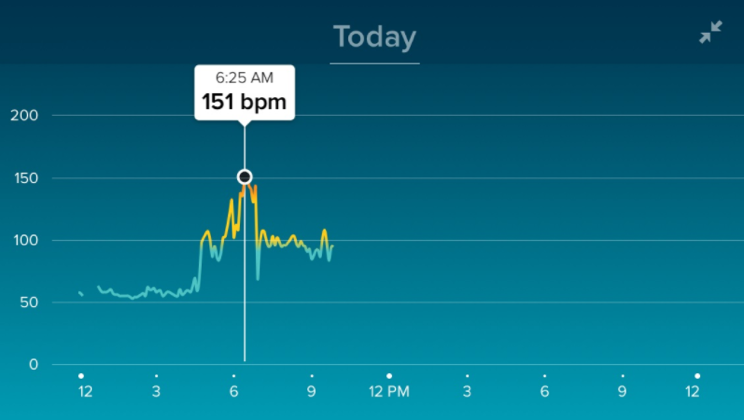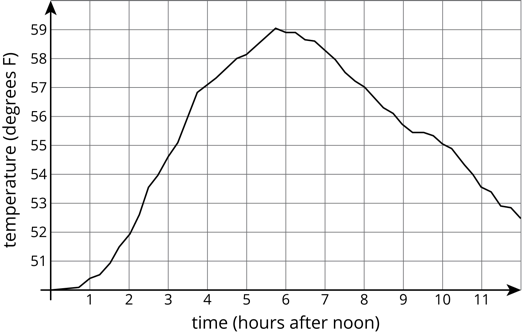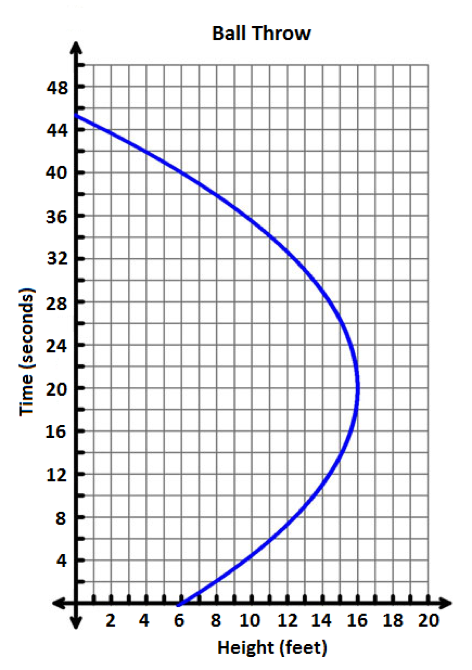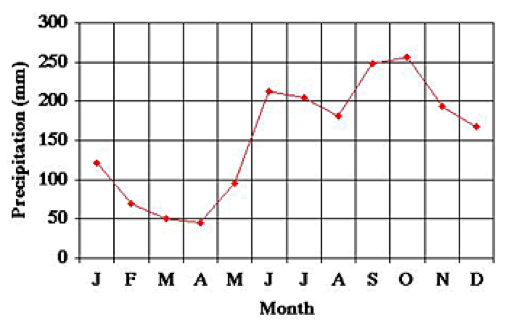# Functions

## Objective

Read inputs and outputs in graphs of functions. Determine if graphs are functions.

## Common Core Standards

### Core Standards

?

• 8.F.A.1 — Understand that a function is a rule that assigns to each input exactly one output. The graph of a function is the set of ordered pairs consisting of an input and the corresponding output. Function notation is not required in Grade 8.

?

• 7.RP.A.2.D

## Criteria for Success

?

1. Identify outputs in a graph given input values.
2. Identify inputs in a graph given output values.
3. Determine if a graph is a function (i.e., if every input value has exactly one output value).
4. Interpret inputs and outputs of graphs in context (MP.2).
5. Create a table of values for a graph.

## Tips for Teachers

?

• Lessons 5 and 6 introduce the graphical representation of functions. In Lesson 5, students analyze graphs to identify inputs and outputs and then determine if one variable is a function of the other.
• In Lesson 6, students will investigate properties of functions as seen in graphs, including rate of change and initial value.

#### Fishtank Plus

• Problem Set
• Student Handout Editor
• Vocabulary Package

## Anchor Problems

?

### Problem 1

Which graph doesn’t belong? Why?#### References

Open Up Resources Grade 8 Unit 5 Lesson 55.1

### Problem 2

A Fitbit is a device that can track your heart rate in beats per minute. The graph below shows Ernest’s heart rate for part of the day, with the number of beats per minute on the $y$-axis and the time of day on the $x$-axis. Use the graph to answer the questions that follow.1. Estimate Ernest’s heart rate at 3 AM and at 9 AM. Explain how you find your answers.
2. At what time(s) in the day was Ernest’s heart rate around 75 beats per minute? Explain how you find your answer(s).
3. What time do you think Ernest woke up this morning?
4. Based on the graph, does heart rate seem to be a function of time of day? Why or why not?
5. Based on this graph, does time of day seem to be a function of heart rate? Why or why not?

#### References

Fitbit Community Photo: Detailed heart rate graph by FerdinandFitbit (Fitbit Community)

### Problem 3

For each graph, create a table of values that includes some of the inputs and outputs. Then use the graph and the table to determine if the relationship is a function.

1.1.

​​​​​​​#### References

Open Up Resources Photo: Grade 8 Unit 5 Lesson 5Graph A from Question 5.2

## Problem Set

?The following resources include problems and activities aligned to the objective of the lesson that can be used to create your own problem set.

• Include spiraled review from first four lessons, asking students to determine if relationships are functions (shown as tables, graphs, verbal descriptions, equations) and to explain why or why not.

?

The graph below shows the average precipitation in a rainforest in Belize over a 12-month period.1. What was the average precipitation in the month of August?
2. In what month(s) was the average precipitation approximately 70 mm?
3. Is precipitation a function of month? Explain why or why not.

#### References

NASA Photo: Temperature and Precipitation Graphs: Rainforest Campa Pita, Belize (NASA Earth Observatory)

Temperature and Precipitation Graphs: Rainforest Campa Pita, Belize by NASA Earth Observatory is made available by NASA. Accessed Oct. 26, 2017, 9:18 p.m..

?